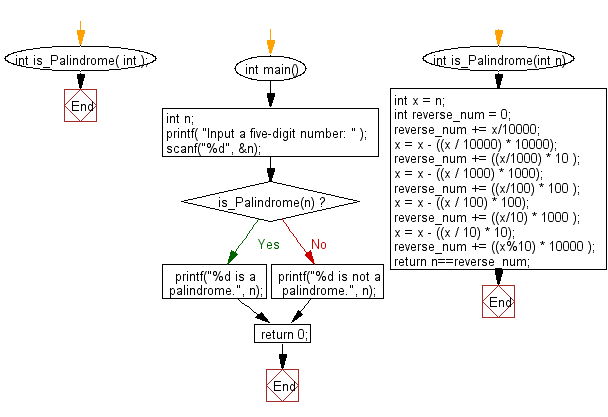﻿ C : Read a 5 digit integer and check if it is a palindrome

# C Exercises: Reads in a five-digit integer and determines whether or not it’s a palindrome

## C Basic Declarations and Expressions: Exercise-82 with Solution

Write a C program that reads a five-digit integer and determines whether or not it's a palindrome.

Sample Input: 33333

Sample Solution:

C Code:

``````#include <stdio.h>

// Function to check if a number is a palindrome
int is_Palindrome(int);

int main()
{
int n;

// Prompt user for input
printf("Input a five-digit number: ");

// Read the number from user
scanf("%d", &n);

// Check if the number is a palindrome using the function is_Palindrome
if (is_Palindrome(n))
printf("%d is a palindrome.", n);
else
printf("%d is not a palindrome.", n);

return 0;
}

// Function to check if a number is a palindrome
int is_Palindrome(int n) {
int x = n;
int reverse_num = 0;

// Extract the digits in reverse order and reconstruct the number
reverse_num += x / 10000;
x = x - ((x / 10000) * 10000);

reverse_num += ((x / 1000) * 10);
x = x - ((x / 1000) * 1000);

reverse_num += ((x / 100) * 100);
x = x - ((x / 100) * 100);

reverse_num += ((x / 10) * 1000);
x = x - ((x / 10) * 10);

reverse_num += ((x % 10) * 10000);

// Check if the original number is equal to the reversed number
return n == reverse_num;
}
``````

Sample Output:

```Input a five-digit number: 33333 is a palindrome.
```

Pictorial Presentation:Flowchart:C programming Code Editor:

What is the difficulty level of this exercise?

Test your Programming skills with w3resource's quiz.

﻿